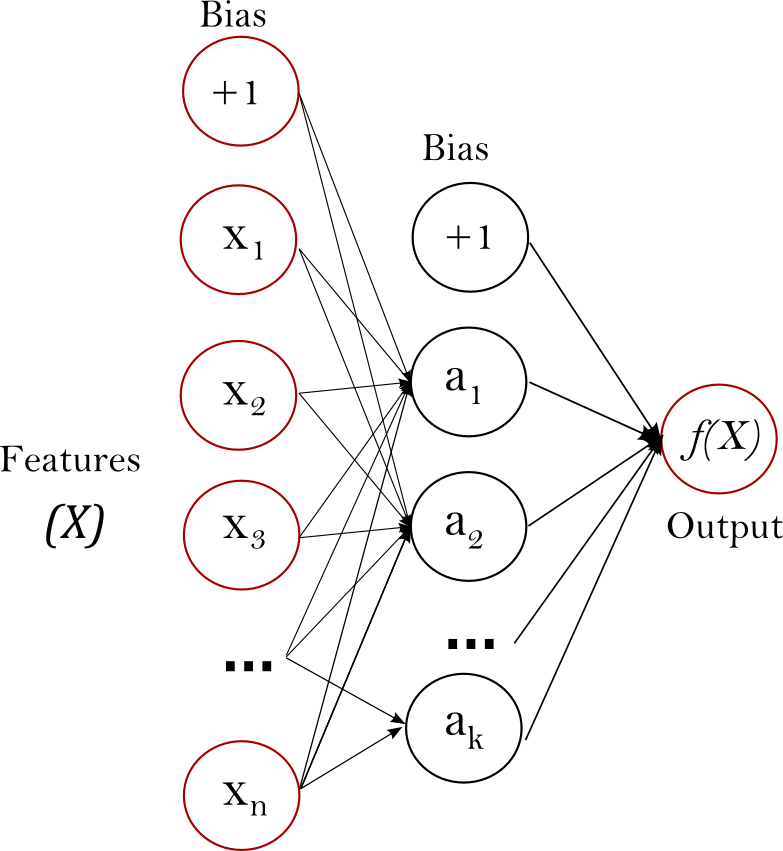# 1.17. 神经网络模型（有监督）

## 1.17.1. 多层感知器• 能够学习非线性模型
• 能够使用partial_fit学到实时(real-time)模型

• 具有隐藏层的MLP具有非凸损失函数，其存在多个局部极小值。因此，不同的随机权重初始化会导致不同的验证精度。
• MLP需要调整许多超参数，例如隐藏层神经元的数量、层数和迭代次数。
• MLP对特征缩放很敏感。

## 1.17.2. 分类

MLPClassifier实现了一个多层感知器（MLP）算法，该算法使用Backpropagation进行训练。

MLP在两个数组上进行训练：大小为(n_samples，n_features)的数组X，它保存浮点型特征向量的训练样本；大小为(n_samples)的数组y，它保存训练样本的目标值（类标签）：

>>> from sklearn.neural_network import MLPClassifier
>>> X = [[0., 0.], [1., 1.]]
>>> y = [0, 1]
>>> clf = MLPClassifier(solver='lbfgs', alpha=1e-5,
...                     hidden_layer_sizes=(5, 2), random_state=1)
...
>>> clf.fit(X, y)
MLPClassifier(alpha=1e-05, hidden_layer_sizes=(5, 2), random_state=1,
solver='lbfgs')


>>> clf.predict([[2., 2.], [-1., -2.]])
array([1, 0])


MLP可以为训练数据拟合一个非线性模型。clf.coefs_包含构成模型参数的权重矩阵：

>>> [coef.shape for coef in clf.coefs_]
[(2, 5), (5, 2), (2, 1)]


MLP使用反向传播进行模型训练。更准确地说，它使用梯度下降来训练，而梯度是用反向传播来计算的。对于分类，它最小化了交叉熵损失函数，给出了每个样本$x$的概率估计向量$P（y | x）$：

>>> clf.predict_proba([[2., 2.], [1., 2.]])
array([[1.967...e-04, 9.998...-01],
[1.967...e-04, 9.998...-01]])


MLPClassifier通过将Softmax作为输出函数来支持多分类。

>>> X = [[0., 0.], [1., 1.]]
>>> y = [[0, 1], [1, 1]]
>>> clf = MLPClassifier(solver='lbfgs', alpha=1e-5,
...                     hidden_layer_sizes=(15,), random_state=1)
...
>>> clf.fit(X, y)
MLPClassifier(alpha=1e-05, hidden_layer_sizes=(15,), random_state=1,
solver='lbfgs')
>>> clf.predict([[1., 2.]])
array([[1, 1]])
>>> clf.predict([[0., 0.]])
array([[0, 1]])


## 1.17.3. 回归

MLPRegressor实现了一个多层感知器（MLP），该感知器使用反向传播进行训练，但在输出层中没有使用激活函数，这也可以看作使用标识函数(identity function)作为激活函数。因此，它使用平方误差作为损失函数，输出是一组连续值。

MLPRegressor 还支持多输出回归，其中一个样本可以有多个目标值。

## 1.17.4. 正则化

MLPRegressorMLPClassifier都使用参数alpha作为正则化（L2正则化）项，这有助于通过惩罚值较大的权重以避免过拟合。下图显示了不同 alpha 值下决策函数的变换。## 1.17.5. 算法

L-BFGS是一个近似Hessian矩阵的求解器，其中，Hessian矩阵表示函数的二阶偏导数。此外，L-BFGS近似Hessian矩阵的逆矩阵以进行参数更新。实现使用了L-BFGS的Scipy版本。

## 1.17.7. 数学公式

MLP根据问题类型使用不同的损失函数。分类使用交叉熵作为损失函数，在二分类情况下，损失函数为， $$Loss(\hat{y},y,W) = -y \ln {\hat{y}} - (1-y) \ln{(1-\hat{y})} + \alpha ||W||_2^2$$ 其中$\alpha ||W||_2^2$是惩罚复杂模型的L2正则化项（aka惩罚）； $\alpha > 0$是控制惩罚大小的非负超参数。

## 1.17.8. 实用小贴士

• 多层感知器对特征缩放敏感，因此强烈建议将数据进行归一化。例如，将输入向量X上的每个属性缩放为[0，1]或[-1，+1]，或将其标准化为平均值0和方差1。请注意，必须对测试集应用相同的缩放比例才能获得有意义的结果。可以使用 StandardScaler进行标准化。
   >>> from sklearn.preprocessing import StandardScaler  # doctest: +SKIP
>>> scaler = StandardScaler()  # doctest: +SKIP
>>> # 不要作弊-只在训练数据集上进行拟合
>>> scaler.fit(X_train)  # doctest: +SKIP
>>> X_train = scaler.transform(X_train)  # doctest: +SKIP
>>> # 对测试数据应用相同的转换
>>> X_test = scaler.transform(X_test)  # doctest: +SKIP


• 最好使用GridSearchCV找到一个合理的正则化参数$\alpha$，通常在 10.0 ** -np.arange(1, 7)范围内。

• 根据经验，我们观察到L-BFGS收敛更快，且在小数据集上具有更好的解。然而，对于相对较大的数据集，Adam鲁棒性强。它通常收敛得很快，性能也很好。另一方面，如果学习速率得到正确的调整，使用带momentum 或 nesterov’s momentum的SGD算法可以比这两种算法有更好的性能。

## 1.17.9. 使用 warm_start 进行更多控制

>>> X = [[0., 0.], [1., 1.]]
>>> y = [0, 1]
>>> clf = MLPClassifier(hidden_layer_sizes=(15,), random_state=1, max_iter=1, warm_start=True)
>>> for i in range(10):
...     clf.fit(X, y)
...     # additional monitoring / inspection
MLPClassifier(...


(C) 2007 - 2019, scikit-learn 开发人员 (BSD 许可证). 此页显示源码